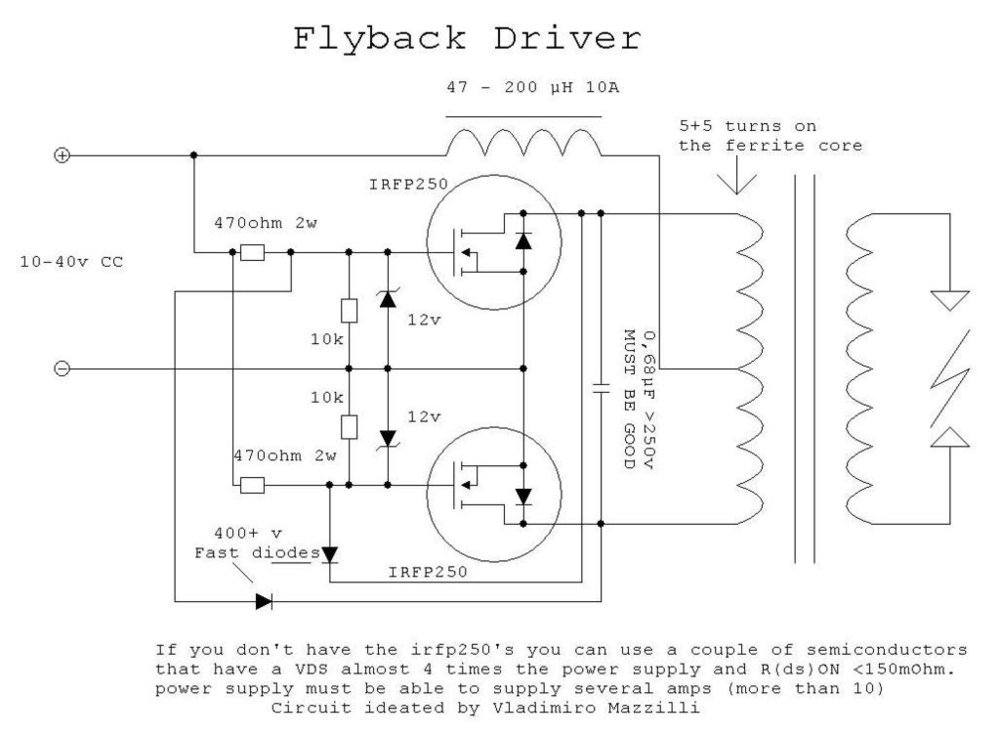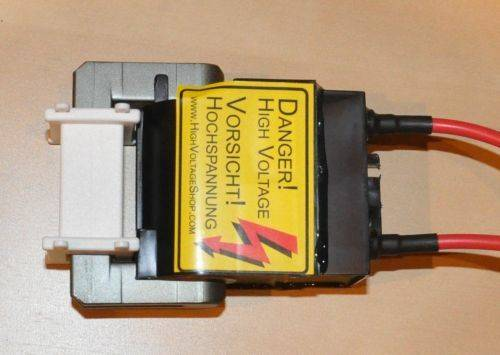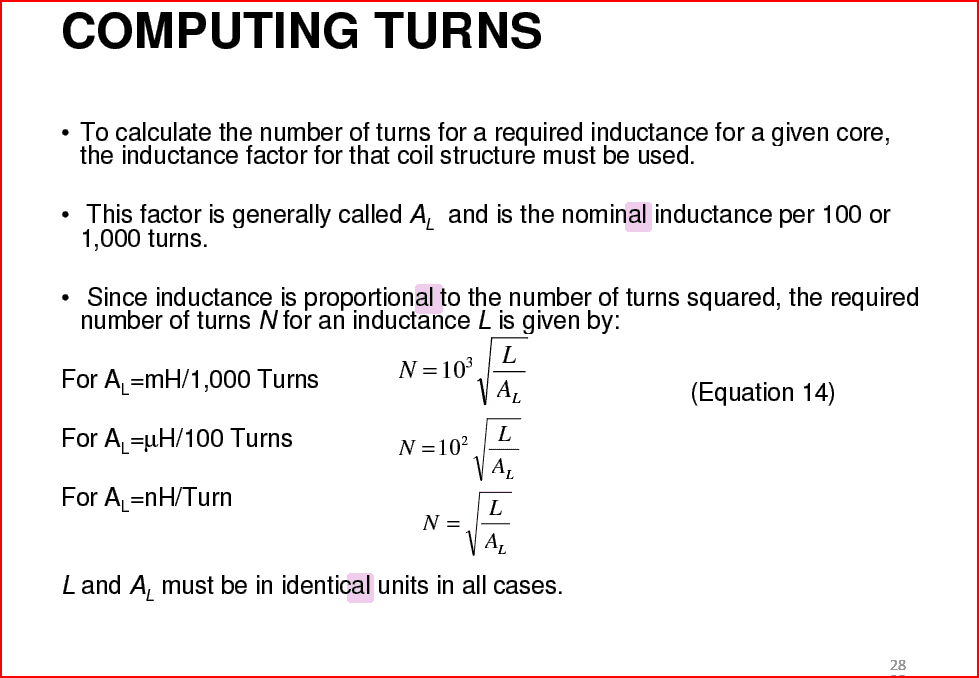# How to calculate the inductance of a center tapped solenoid ?

I'm trying to drive a transformer using a ZVS power supply. The primary coil that we use here has to be wound center tapped. The output frequency depends on the inductance of the primary winding and capacitance used in the circuit.
The circuit of a ZVS power supply is as follows,The transformer where the primary will be wound is as follows. It's a flyback transformer where the secondary is present and primary can be wound as we need.berkeman
Mentor
Do you have the A_l (A-sub-l) value for that core?

What's A_I value means ? Is it the core permeability value ?

berkeman
Mentor
What's A_I value means ? Is it the core permeability value ?
It is usually given for cores so that you can compute the inductance based on the number of turns. Look at the datasheet for your core, for example.

This is a pretty good introduction to inductor design, and it includes a discussion of A_L:

https://www.ieee.li/pdf/viewgraphs/fundamentals_magnetics_design.pdfOkay. Is there any thing that I need to take into account since it's a center tapped inductor ? Unlike 2 terminal inductors this one has 3 terminals due to center tap.

berkeman
Mentor
But only one of the two primary coils is energized at any given time, right?

•zoki85